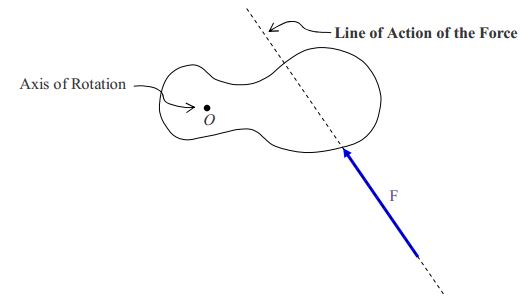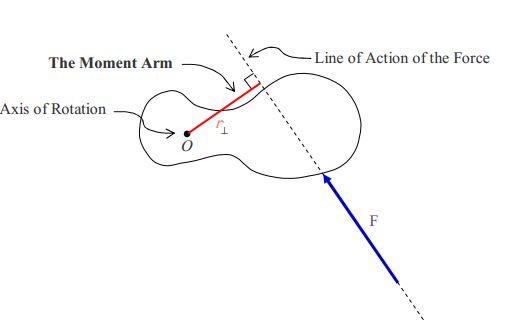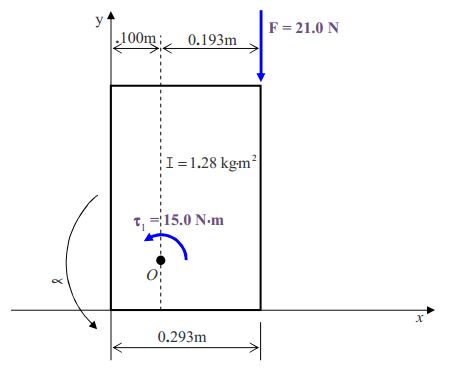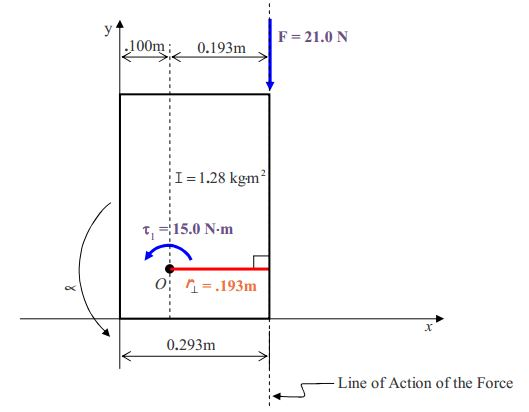$$\require{cancel}$$

# 20A: Torque & Circular Motion

The mistake that crops up in the application of Newton’s 2nd Law for Rotational Motion involves the replacement of the sum of the torques about some particular axis, $$\sum \tau_{o\circlearrowleft}$$, with a sum of terms that are not all torques. Oftentimes, the errant sum will include forces with no moment arms (a force times a moment arm is a torque, but a force by itself is not a torque) and in other cases the errant sum will include a term consisting of a torque times a moment arm (a torque is already a torque, multiplying it by a moment arm yields something that is not a torque). Folks that are in the habit of checking units will catch their mistake as soon as they plug in values with units and evaluate.

We have studied the motion of spinning objects without any discussion of torque. It is time to address the link between torque and rotational motion. First, let’s review the link between force and translational motion. (Translational motion has to do with the motion of a particle through space. This is the ordinary motion that you’ve worked with quite a bit. Until we started talking about rotational motion we called translational motion “motion.” Now, to distinguish it from rotational motion, we call it translational motion.) The real answer to the question of what causes motion to persist, is nothing—a moving particle with no force on it keeps on moving at constant velocity. However, whenever the velocity of the particle is changing, there is a force. The direct link between force and motion is a relation between force and acceleration. The relation is known as Newton’s 2nd Law of Motion which we have written as equation $$\ref{14-1}$$:

$\vec{a}=\frac{1}{m} \sum \vec{F}$

in which,

• $$\vec{a}$$ $$\space$$ is the acceleration of the object, how fast and which way its velocity is changing
• $$m$$ $$\space$$ is the mass, a.k.a. inertia, of the object. $$\frac{1}{m}$$ can be viewed as a sluggishness factor, the bigger the mass $$m$$, the smaller the value of $$\frac{1}{m}$$ and hence the smaller the acceleration of the object for a given net force. (“Net” in this context just means “total”.)
• $$\sum\vec{F}$$ $$\space$$ is the vector sum of the forces acting on the object, the net force.

We find a completely analogous situation in the case of rotational motion. The link in the case of rotational motion is between the angular acceleration of a rigid body and the torque being exerted on that rigid body.

$\vec{\alpha}=\frac{1}{I} \sum \vec{\tau} \label{20-1}$

in which,

• $$\vec{\alpha}$$ is the angular acceleration of the rigid body, how fast and which way the angular velocity is changing
• $$I$$ is the moment of inertia, a.k.a. the rotational inertia (but not just plain old inertia, which is mass) of the rigid body. It is the rigid body’s inherent resistance to a change in how fast it (the rigid body) is spinning. (“Inherent” means “of itself”, “part of its own being.”) $$\frac{1}{I}$$ can be viewed as a sluggishness factor, the bigger the rotational inertia $$I$$, the smaller the value of $$\frac{1}{I}$$ and hence the smaller the angular acceleration of the object for a given net torque.
• $$\sum\vec{\tau}$$ is the net torque acting on the object. (A torque is what you are applying to a bottle cap or jar lid when you are trying to unscrew it.)

# The Vector Nature of Torque and Angular Velocity

You’ve surely noticed the arrows over the letters used to represent torque, angular acceleration, and angular velocity; and as you know, the arrows mean that the quantities in question are vector quantities. That means that they have both magnitude and direction. Some explanation about the direction part is in order. Let’s start with the torque. As mentioned, it is a twisting action such as that which you apply to bottle cap to loosen or tighten the bottle cap. There are two ways to specify the direction associated with torque. One way is to identify the axis of rotation about which the torque is being applied, then to establish a viewpoint, a position on the axis, at a location that is in one direction or the other direction away from the object. Then either state that the torque is clockwise, or state that it is counterclockwise, as viewed from the specified viewpoint. Note that it is not sufficient to identify the axis and state “clockwise” or “counterclockwise” without giving the viewpoint—a torque which is clockwise from one of the two viewpoints is counterclockwise from the other. The second method of specifying the direction is to give the torque vector direction. The convention for the torque vector is that the axis of rotation is the line on which the torque vector lies, and the direction is in accord with “the right hand rule for something curly something straight.”

By the “the right hand rule for something curly something straight”, if you point the thumb of your cupped right hand in the direction of the torque vector, the fingers will be curled around in that direction which corresponds to the sense of rotation (counterclockwise as viewed from the head of the torque vector looking back along the shaft) of the torque.The angular acceleration vector $$\vec{\propto}$$ and the angular velocity vector $$\vec{\omega}$$ obey the same convention. These vectors, which point along the axis about which the rotation they represent occurs, are referred to as axial vectors.

# The Torque Due to a Force

When you apply a force to a rigid body, you are typically applying a torque to that rigid body at the same time. Consider an object that is free to rotate about a fixed axis. We depict the object as viewed from a position on the axis, some distance away from the object. From that viewpoint, the axis looks like a dot. We give the name “point O” to the position at which the axis of rotation appears in the diagram and label it “O” in the diagram to make it easier to refer to later in this discussion. There is a force $$\vec{F}$$ acting on the object.The magnitude of the torque due to a force is the magnitude of the force times the moment arm $$r_{\bot}$$ (read “r perp”) of the force. The moment arm (r_{\bot}\) is the perpendicular distance from the axis of rotation to the line of action of the force. The line of action of the force is a line that contains the force vector. Here we redraw the given diagram with the line of action of the force drawn in.Next we extend a line segment from the axis of rotation to the line of action of the force, in such a manner that it meets the line of action of the force at right angles. The length of this line segment is the moment arm $$r_{\bot}$$.The magnitude of the torque about the specified axis of rotation is just the product of the moment arm and the force.

$\tau=r_{\bot} F \label{20-2}$

# Applying Newton’s Second Law for Rotational Motion in Cases Involving a Fixed Axis

Starting on the next page, we tell you what steps are required (and what diagram is required) in the solution of a fixed-axis “Newton’s 2nd Law for Rotational Motion” problem by means of an example

Example 20-1:

A flat metal rectangular 293 mm $$\times$$ 452 mm plate lies on a flat horizontal frictionless surface with (at the instant in question) one corner at the origin of an x-y coordinate system and the opposite corner at point P which is at (293 mm, 452 mm). The plate is pin connected to the horizontal surface at (10.0 cm, 10.0 cm). A counterclockwise (as viewed from above) torque, with respect to the pin, of 15.0 N$$\cdot$$m, is being applied to the plate and a force of 21.0 N in the –y direction is applied to the corner of the plate at point $$P$$. The moment of inertia of the plate, with respect to the pin, is 1.28 $$kg \cdot m^2$$. Find the angular acceleration of the plate at the instant for which the specified conditions prevail.

We start by drawing a pseudo free body diagram of the object as viewed from above (downward is “into the page”):We refer to the diagram as a pseudo free body diagram rather than a free body diagram because:

a. We omit forces that are parallel to the axis of rotation (because they do not affect the rotation of the object about the axis of rotation). In the case at hand, we have omitted the force exerted on the plate by the gravitational field of the earth (which would be “into the page” in the diagram) as well as the normal force exerted by the frictionless surface on the plate (“out of the page”).

b. We ignore forces exerted on the plate by the pin. (Such forces have no moment arm and hence do not affect the rotation of the plate about the axis of rotation. Note, a pin can, however, exert a frictional torque—assume it to be zero unless otherwise specified.)

Next we annotate the pseudo free body diagram to facilitate the calculation of the torque due to the force F:Now we go ahead and apply Newton’s 2nd Law for Rotational Motion, equation $$\ref{20-1}$$ :

$\vec{\propto}= \frac{1}{I} \sum\vec{\tau}$

As in the case of Newton’s 2nd Law (for translational motion) this equation is three scalar equations in one, one equation for each of three mutually perpendicular axes about which rotation, under the most general circumstances, could occur. In the case at hand, the object is constrained to allow rotation about a single axis. In our solution, we need to indicate that we are summing torques about that axis, and we need to indicate which of the two possible rotational senses we are defining to be positive. We do that by means of the subscript $$\circ_{\circlearrowleft}$$ to be read “counterclockwise about point $$O$$.” Newton’s 2nd Law for rotational motion about the vertical axis (perpendicular to the page and represented by the dot labeled “$$O$$” in the diagram) reads:

$\propto_{O_{\circlearrowleft}}=\frac{1}{I}\sum\tau_{O_{\circlearrowleft}} \label{20-3}$

Now, when we replace the expression $$\frac{1}{I}\sum\tau_{O_{\circlearrowleft}}$$ with the actual term-by-term sum of the torques, we note that $$\tau_1$$ is indeed counterclockwise as viewed from above (and hence positive) but that the force $$\vec{F}$$, where it is applied, would tend to cause a clockwise rotation of the plate, meaning
that the torque associated with force $$\vec{F}$$ is clockwise and hence, must enter the sum with a minus sign.

$\propto= \frac{1}{I}(\tau_1-r_{\bot}F$

Substituting values with units yields:

$\propto=\frac{1}{1.28kg\cdot m^2} \Big [15.0N\cdot m-0.193m(21.0N)\Big]$

Evaluating and rounding the answer to three significant figures gives us the final answer:

$\propto=8.55 \frac{\mbox{rad}}{s^2} \mbox{(counterclockwise as viewed from above)}$

Regarding the units we have:

$\frac{1}{kg\cdot m^2} N \cdot m= \frac{1}{kg \cdot m} \frac{kg \cdot m}{s^2} =\frac{1}{s^2} =\frac{\mbox{rad}}{s^2}$

where we have taken advantage of the fact that a newton is a $$\frac{kg \cdot m}{s^2}$$ and the fact that the radian is not a true unit but rather a marker that can be inserted as needed.